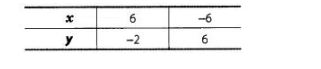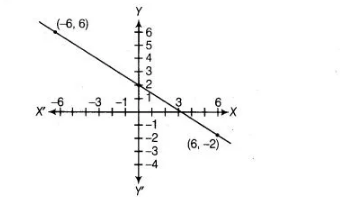# The following observed values of`
Question:

The following observed values of $x$ and $y$ are thought to satisfy a linear equation. Write the linear equationDraw the graph, using the values of x, y as given in the above table. At what points the graph of the linear equation

(i) cuts the X-axis?

(ii) cuts the Y- axis?

Solution:

Given, points are $(6,-2)$ and $(-6,6)$.

Let the linear equation $y=m x+c$ is satisfied by the points $(6,-2)$ and $(-6,6)$, then at point $(6,-2)$

$-2=6 m+c$$\ldots$ (i)

and at point $(-6,6)$,

$6=-6 m+c$ .....(ii)

On subtracting Eq. (ii) from Eq. (i), we get

$12 m=-8 \Rightarrow m=\frac{-8}{12} \Rightarrow m=-\frac{2}{3}$

On putting the value of $m$ in Eq. (i), we get

$-2=6(-2 / 3)+c$

$\Rightarrow$ $-2=-4+c \Rightarrow c=-2+4 \Rightarrow c=2$

On putting $m=-\frac{2}{3}$ and $c=2$ in linear equation $y=m x+c$ we get$y=-\frac{2}{3} x+2$

$\Rightarrow$ $y=\frac{-2 x+6}{3}$

$\Rightarrow$ $3 y=-2 x+6$

$\Rightarrow$ $3 y+2 x=6$

When the graph of the linear equation

(i) Cuts the $X$-axis

Then, put $y=0$ in equation $2 x+3 y=6$. we get

$2 x+3 \cdot 0=6$

$\Rightarrow \quad 2 x=6$

$\therefore \quad x=3$

When the graph of the linear equation

\text { (ii) Cuts the Y-axis }

Then, put $x=0$ in equation $2 x+3 y=6$, we get

$\Rightarrow \quad 2 \cdot 0+3 y=6$

$\Rightarrow \quad 3 y=6$

$\therefore \quad y=2$

Therefore, the graph the linear equation cuts the $X$-axis at the point $(3,0)$ and the $Y$-axis at the point $(0,2)$.